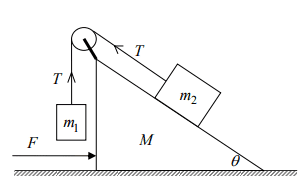# Newton's laws: Block/Pulley/Wedge/Friction

## Homework StatementNo friction between m2 and M.
The static friction between M and the floor is μs and the dynamic is μd.
When F=0, what is the minimal static friction if M doesn't move?

## Homework Equations

F=ma
Force caused by friction: μN=F

## The Attempt at a Solution

What I thought is that m2 is affected by gravity, normal and tension and that M is affected by m2's normal, gravity, it's own normal and the friction.
I wrote the following equations:
N=m2gcosθ
Ncosθ+Mg=N' (N' is M's normal).
Nsinθ=μsN'
μs=m2gsinθcosθ/(Mg+m2gcos2θ)What am I missing here?

#### Attachments

haruspex
Homework Helper
Gold Member
2020 Award
What am I missing here?
The forces exerted on the pulley (and thus on M) by the rope.

The forces exerted on the pulley (and thus on M) by the rope.
Thanks!!

The forces exerted on the pulley (and thus on M) by the rope.
I still don't get the correct answer, is my algebra the problem or my equations:
m1a=m1g-T
m2a=T-m2gsinθ
N=m2gcosθ
N'=Ncosθ+Mg+(1+sinθ)T
Tcosθ=μsN'+Nsinθ

haruspex
Homework Helper
Gold Member
2020 Award
I still don't get the correct answer, is my algebra the problem or my equations:
m1a=m1g-T
m2a=T-m2gsinθ
N=m2gcosθ
N'=Ncosθ+Mg+(1+sinθ)T
Tcosθ=μsN'+Nsinθ
Is it obvious which way friction will act?

Is it obvious which way friction will act?
No, but I have no idea how to check it.

haruspex
Homework Helper
Gold Member
2020 Award
No, but I have no idea how to check it.
The official answer seems to have made an assumption. There is a factor in the numerator that could in principle be negative, so they must be assuming it is positive. Or is there some information you have left out?
Your equations in post #4 make the opposite assumption.

The official answer seems to have made an assumption. There is a factor in the numerator that could in principle be negative, so they must be assuming it is positive. Or is there some information you have left out?
Your equations in post #4 make the opposite assumption.
The exact words:
"A force equals to F applies on a surface with a mass of M. The dynamic and static friction coefficients of M with the floor are μD and μS respectively. A body with a mass of m1 is connected with a pulley to another body with a mass of m2, which slides without friction on M.
First they asked what is the acceleration of m2 assuming μs is very big, but this was not mentioned in this part of the question so I thought I am not allowed to use this. Does this resolve it?

haruspex
Homework Helper
Gold Member
2020 Award
Does this resolve it?
Not really. The trouble is that we cannot tell which way the masses will move.
If m1 > m2 sin(θ) then m1 descends and m2 slides left. That will tend to push M right, so friction acts to the left. This seems to be the problem setter's intent. But in your equations you have taken friction as acting to the right, which would be correct if m1 < m2 sin(θ).
I cannot tell if you had any algebraic errors beyond that.

Another clue to the intent is the force F that comes in later.

Not really. The trouble is that we cannot tell which way the masses will move.
If m1 > m2 sin(θ) then m1 descends and m2 slides left. That will tend to push M right, so friction acts to the left. This seems to be the problem setter's intent. But in your equations you have taken friction as acting to the right, which would be correct if m1 < m2 sin(θ).
I cannot tell if you had any algebraic errors beyond that.

Another clue to the intent is the force F that comes in later.

That was my problem as well.
So basically, it's impossible to determine this?

haruspex
Homework Helper
Gold Member
2020 Award
That was my problem as well.
So basically, it's impossible to determine this?
I am saying that the problem setter forgot to specify that m1 > m2 sin(θ). So assume that and try again. You will need to change your last equation in post #4.

•Eitan Levy
I am saying that the problem setter forgot to specify that m1 > m2 sin(θ). So assume that and try again. You will need to change your last equation in post #4.
Alright, thank you.

TSny
Homework Helper
Gold Member
The given answer should not have a factor of g in the numerator.

By taking the absolute value of the numerator, the answer will be valid for all values of ##m_1## and ##m_2##.